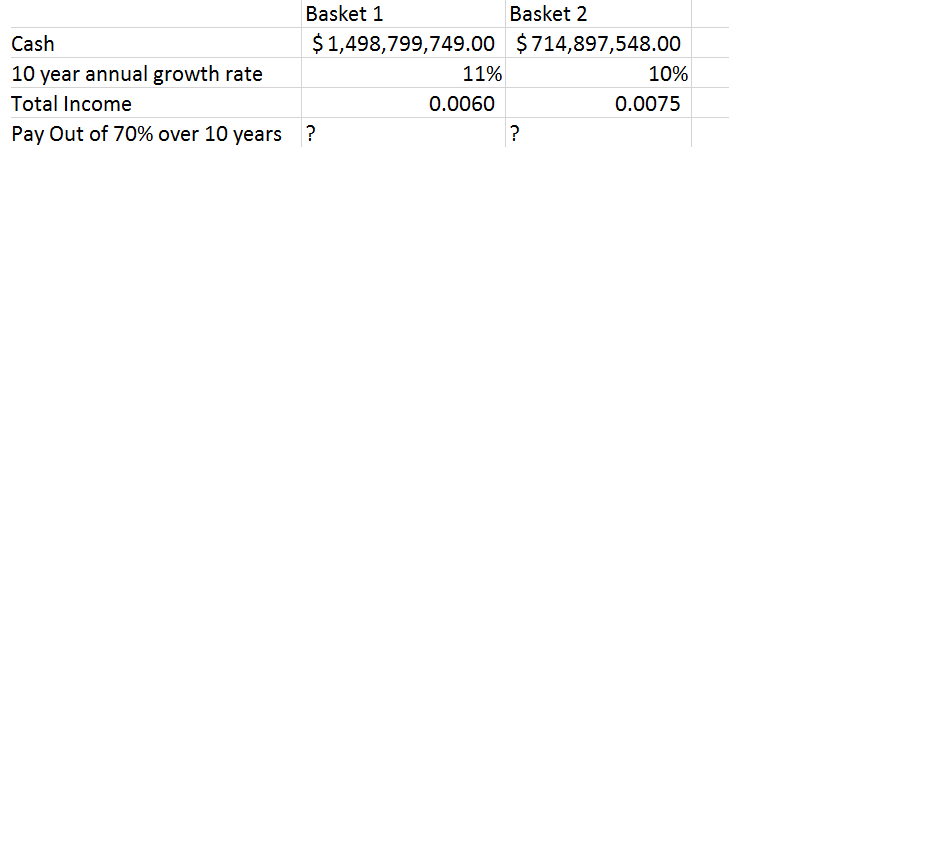### Create an Account

Home / Questions / Income section is the fee charges on the cash amount Calculate the Present Value for each ...

# Income section is the fee charges on the cash amount Calculate the Present Value for each basket over a 10 year period at 11% growth rate and 10% growth rate for each I have fund #1 which I want to

Income section is the fee charges on the cash amount. Calculate the Present Value for each basket over a 10 year period  at 11% growth rate and 10% growth rate for each.I have fund #1 which I want to calculate the present value for 10 years and then calculate how much i am making at .0060%.Aug 16 2020 View more View LessSubscribe To Get Solution